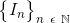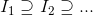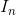Introduction to Analysis : Sequences in Real Numbers (R)

Example Questions

Example Question #1 : Sequences In Real Numbers (R)

What term does the following define.

A sequence of setsis __________ if and only if.

Increasing

Unbounded

Nested

Decreasing

Bounded

Nested

Explanation:

This statement:

A sequence of setsis __________ if and only ifis the definition of nested.

This means that the sequencefor allelements, for whichbelongs to the natural numbers, is considered a nested set if and only if the subsequent sets are subsets of it.

Other theorems in intro analysis build off this understanding.